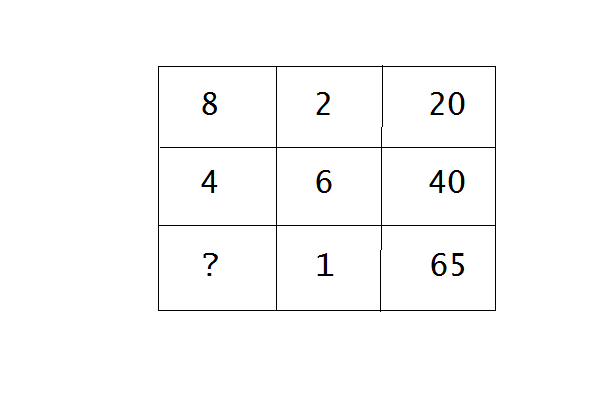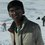# Confusing MAT ProblemOpen the link given above and see the question mark. Please fill in the question mark. There is a pattern either row wise or column wise. If someone wants the options, they are posted below: (A)14 (B)15 (C)16 (D)17Note by Vinayak Verma
6 years, 9 months ago

This discussion board is a place to discuss our Daily Challenges and the math and science related to those challenges. Explanations are more than just a solution — they should explain the steps and thinking strategies that you used to obtain the solution. Comments should further the discussion of math and science.

When posting on Brilliant:

• Use the emojis to react to an explanation, whether you're congratulating a job well done , or just really confused .
• Ask specific questions about the challenge or the steps in somebody's explanation. Well-posed questions can add a lot to the discussion, but posting "I don't understand!" doesn't help anyone.
• Try to contribute something new to the discussion, whether it is an extension, generalization or other idea related to the challenge.

MarkdownAppears as
*italics* or _italics_ italics
**bold** or __bold__ bold
- bulleted- list
• bulleted
• list
1. numbered2. list
1. numbered
2. list
Note: you must add a full line of space before and after lists for them to show up correctly
paragraph 1paragraph 2

paragraph 1

paragraph 2

[example link](https://brilliant.org)example link
> This is a quote
This is a quote
    # I indented these lines
# 4 spaces, and now they show
# up as a code block.

print "hello world"
# I indented these lines
# 4 spaces, and now they show
# up as a code block.

print "hello world"
MathAppears as
Remember to wrap math in $$ ... $$ or $ ... $ to ensure proper formatting.
2 \times 3 $2 \times 3$
2^{34} $2^{34}$
a_{i-1} $a_{i-1}$
\frac{2}{3} $\frac{2}{3}$
\sqrt{2} $\sqrt{2}$
\sum_{i=1}^3 $\sum_{i=1}^3$
\sin \theta $\sin \theta$
\boxed{123} $\boxed{123}$

Sort by:

Well guys, I think Rashmi gave the most accurate answer of this problem. Thanks a lot Rashmi. Nice try Ivan, Bhargav and Likhita!

- 6 years, 9 months ago

I think the logic used here ,to get one of the options given as the answer is: $20 - (8 \times 2)=4=2^{2}$ $40 - (4 \times 6)=16=4^{2}$. That is, the above pattern is followed to get a perfect square. So,putting the various values we get,$16$ as our required answer as $65 - (1 \times 16)=49=7^{2}$, which is a perfect square. Hope that helps!

- 6 years, 9 months ago

I tried this logic of yours many a times but I don't think it is the most accurate answer. Nonetheless, nice try Bhargav. I think Rashmi gave the most accurate answer. Go check hers!

- 6 years, 9 months ago

i got 64 without the the options

- 6 years, 9 months ago

In the examination, the options enlisted were only these. I am sure the answer is one of the above options which I gave. Thank You for giving an answer. Can you provide a solution also?

- 6 years, 9 months ago

As stated, this is not a magic square. But assuming it's one of those "brain "teaser" tests", here's a solution that I see perfectly valid while not helping you at all:

The third number in each row is the minimum of the other two numbers in the row, multiplied by their sum.

By this, the question mark is either $64$ or $\dfrac{-1-3\sqrt{29}}{2}$. Neither is among the given choices.

The above simply states that doing such questions just wastes your time, because there are infinitely many interpretations possible, and a bunch of "plausible" interpretations.

- 6 years, 9 months ago

Thank you Ivan for correcting me! The same question appeared in an examination which I gave. Can you come up with a solution among the four options?

- 6 years, 9 months ago

This is not a magic square ! The sum of the numbers in each column and row must be same in a Magic square

- 6 years, 9 months ago

Thank You for correcting my knowledge about the magic squares. Well, did you come up with any more answers?

- 6 years, 9 months ago

$8+2 + 10 = 20$

$4+6+ 30 = 40$

$x+1+ 50 = 65$

we end up $x=14$

- 6 years, 9 months ago

I think you are somewhat correct as you got the most possibly correct answer. Thanks a ton. Cheers!

- 6 years, 9 months ago